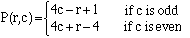Subject: Finding a formula

Name: Erica Hildebrandt Who is asking: Other Level: All

Question:
If a farmer has a field and his plots are laid out in the following grid where each # represents a plot:

 4 5 12 13 20 3 6 11 14 19 2 7 10 15 18 1 8 9 16 17

Of course the plot numbers aren't meaningful as I have described above. In fact they may not be numbers at all. The only constants I have are the total number of rows and columns. Using the total number of rows and columns and my current position row and column, how can I write a formula that tells me column 3 row 3 = 10, column 4 row 2 = 14, etc. I can see the pattern but can't quite get the formula. I believe I will need 2 different formulas one for even and one for odd rows. Any help is greatly appreciated!!

Thanks!!

Erica

Hi Erica,

I want to use the notation P(r,c) where P is the plot number, r is the row number and c is the column number. Thus, as you said in your question P(3,3) = 10 and P(2,4) = 14.

In each column the largest plot number is 4c, that is 4 times the column number. In the odd numbered rows the plot number decreases as you move from row 1 to row 2 to row 3 to row 4. Explicitly in each odd numbered column the plot numbers are 4c, 4c - 1, 4c - 2 and 4c - 3. That is for the odd columns P(r,c) = 4c -(r - 1) = 4c - r + 1.

For the even column the plot numbers are 4c - 3, 4c - 2, 4c - 1 and 4c. That is for the even rows the plot numbers are P(r,c) = 4c -(4 - r) = 4c + r - 4.

Hence one way you can write an expression for the plot number isYou can write this as a "one-line" expression if you know the modulo function. If b and m are positive integers then

b mod m is the remainder obtained when b is divided by m.

Thus (7 mod 3) is 1 and (12 mod 4) is 0.

In your example (c mod 2) is 0 if c is even and (c mod 2) is 1 if c is odd. Hence P(r,c) can be written

P(r,c) = (4c + r - 4) + (5 - 2r)(c mod 2)

When c is even P(r,c) = (4c + r - 4) + 0 = 4c + r - 4, and when c is odd P(r,c) = (4c + r - 4) + (5 - 2r)(1) = 4c - r + 1.

Cheers,
Paul and Penny
Go to Math Central